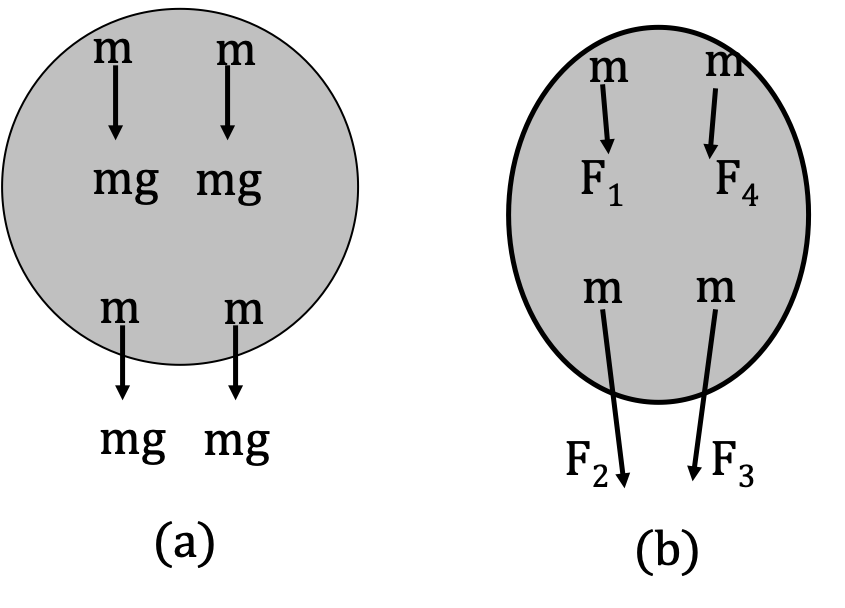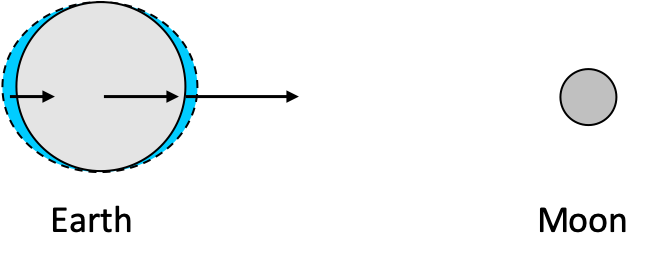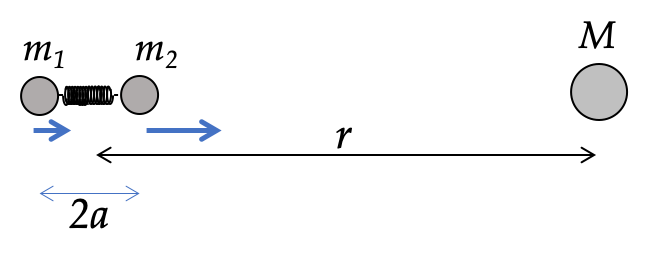## Section12.9Tidal Forces

When a uniform force, such as the approximately constant force of gravity near the Earth of magnitude $mg$ acts on an object, each particle of the object accelerates equally. We do not get a differential force across the body.

Unlike $mg\text{,}$ gravitation force varies over space, and, that can result in a differential force on an extended body. For instance, the force of gravitation on Earth from the Sun is different at different parts of Earth since they are at different distances from the Sun. The force on the side of the Earth that faces the Sun will be larger than the force on the opposite side of the Earth.

When a body is subjected to a non-uniform force, different parts of the body experience different forces, and consequently accelerate to different extent. As a result,a deformable body will be deformed as illustrated in Figure 12.9.1.

On the other hand, if the body is not deformable, then stresses in the body will develop. If the stress inside the body is large enough, the body may break apart as a result of the non-uniform force.Figure 12.9.1. (a) In a uniform gravity, equal forces act on equal masses m of at all parts of the body. (b) Under a non-uniform force, which is the case with the gravitational force, not the approximate force of gravity near the Earth, different parts of a body experience different force, which causes the body to stretch out in the direction of the force.

The deformation of a body or stress in a body subject to a non-uniform force is said to be caused by the differential force that depends on how the force varies over the body. The ocean tides on the Earth are largely caused by the non-uniform gravitational force of the Moon as illustrated inFigure 12.9.2. The ocean tides on the Earth are primarily as a result of the tidal forces from the non-uniform gravitational force of the Moon. The ocean water on the side facing the Moon is pulled towards the Moon due to a stronger force on that side, and at the same time, the solid part of the Earth is pulled towards the Moon and away from the the ocean water on the far side. Every 24 hours, a side of the Earth is either facing towards the Moon or facing away from the Moon, and that is why we have two tides per day. different force, which causes the body to stretch out in the direction of the force.

Due to this association with the ocean tides, the differential forces on a body due to a non-uniform force are also called the tidal forces. Although, the net force of the Sun on the Earth is far greater than the force of the Moon on the Earth, the gradient at which the force of the Moon varies over the Earth is greater than the gradient at which the force of Sun varies on the Earth due to the relative proximity of the Moon.

### Subsection12.9.1(Calculus) Understanding Origin of Tides

To understand the origin of a tidal forces, consider two equal masses $m_1=m$ and $m_2=m$ connected by a spring placed near a large mass $M\text{.}$ Let the separation of the masses be $2a$ and distance from the center of $M$ to the midway between the two masses be $r$ as shown in Figure 12.9.3.Figure 12.9.3. A system of two masses near another mass will have a tidal force on the two mass system.

The differential tidal force on the two-mass system will be the difference of the forces on the two masses by the external body $M\text{.}$

\begin{equation*} |\Delta\vec F| = \frac{GMm}{(r-a)^2}-\frac{GMm}{(r+a)^2}. \end{equation*}

Often, we are interested in situations when $a\lt\lt r\text{.}$ We can try to expand the quantity in the parenthesis in a Maclaurin series for $a/r\text{,}$ and keep the first non-vanishing term.

\begin{equation*} \frac{1}{(r\pm a)^2} = \frac{1}{r^2}\left(1\pm \frac{a}{r} \right)^{-2} = \frac{1}{r^2}\left( 1\mp \frac{2a}{r}+\cdots \right) \end{equation*}

Therefore,

\begin{align*} \frac{1}{(r-a)^2}-\frac{1}{(r+a)^2} \amp = \frac{1}{r^2}\left( 1+\frac{2a}{r}+\cdots \right) - \\ \amp\ \ \ \ \ \ \ \frac{1}{r^2}\left( 1-\frac{2a}{r}+\cdots \right)\\ \amp\approx \frac{4a}{r^3}, \text{ only the leading term.} \end{align*}

Keeping only the leading non-vanishing term yields the following expression for the tidal force in case of $a\lt\lt r\text{.}$

\begin{equation*} |\Delta\vec F| = \frac{4GMma}{r^3}. \end{equation*}

Therefore, the tidal force goes as $1/r^3\text{,}$ which explains why the tidal force by the Moon on the Earth is much larger than that by the Sun, even though the later is much more massive.

The tidal force basically acts as a net repulsive force within the body along the direction of the non-uniform force due to a larger acceleration of one side of the body as compared to the other side. Therefore, if the tidal force is greater than the binding force of the body, the object will break apart.

Naturally, the tidal forces have enormous consequences in Astronomy since the forces in large object dealt in the Astronomy are considerable compared to the binding forces among materials. Astronomical objects, such as stars, planets, moons, and comets are held together by gravitational attraction. For instance, when a two-body system held together by their mutual gravitational attraction comes close to another massive body, the two bodies can be torn apart due to the tidal force from the massive body on the two-body system. There is a critical distance, called the Roche limit after the French physicist Edouard Roche, for this to happen.

The Roche limit of a two mass-system can be worked out by demanding that the tidal force from the external large mass must be greater than the force of attraction between the two masses. Let the two-mass system have equal masses $m$ and be separated by a distance $2a\text{.}$ Let the distance between the center of the two-mass system to the center of an external body of mass $M$ be $r$ as shown in Figure 12.9.3. Then, Roche limit will require that

\begin{align*} \amp |\Delta\vec F|_{\textrm{tidal}} > F_{\textrm{between m's}}\ \ \ \text{(break up condition)} \\ \amp\ \ \ \Longrightarrow \frac{4GMma}{r^3} > \frac{Gmm}{4a^2}\\ \amp\ \ \ \Longrightarrow r \lt a \left[\frac{16M}{m}\right]^{1/3}. \end{align*}

The idea of disintegration of the two-body system shown here can be extended to the disintegration of a single body such as the Earth due to the tidal force from an external body such as the Sun. If the Sun came closer than the Roche limit, also called the Roche radius, the Earth will disintegrate. Sure enough, you don't find any moons within the Roche limits of any planets. The bodies that revolving around the planets, Jupiter, Saturn, Uranus and Neptune within their Roche limits are small rocks forming rings unable to clump into larger masses.